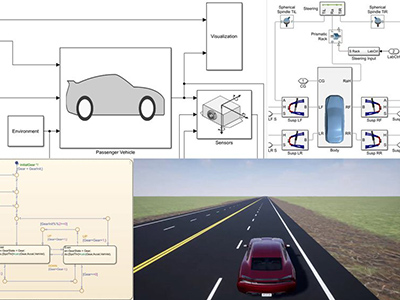ΓραμματείαSecretariat: 2410 684574 | ΦοιτητικάStudents: 2410 684387 g-ds@uth.gr
Select Page

# Systems Design and Simulation

Ε703

7

#### Hours/Week - ECTS

4 – 5### Learning Outcomes

The main objective of this course is to teach students the fundamental concepts of systems simulation using a hands-on approach. Students will be taught the basic concepts of systems simulation, the corresponding models’ validation, and the simulation results’ evaluation. After the introduction, students will be able to use the corresponding simulator to create simulation models.

After attending the course students will be able to:

• Evaluate the advantages and disadvantages of different simulation techniques and choose the appropriate method.
• Be familiar with simulation environments and languages ​​such as MATLAB, ModelSim, OMNET++, etc.
• Design simulation models for simple and complex systems.
• Evaluate statistics and visualize the results of simulations.
• Have the ability to simulate in a fast, flexible, and reliable way applications of future wireless network systems, for example, 5G, wireless vehicle networks, etc.

### Indicative Module Content

Simulation is a technique by which the computer is used for the reliable description (model) and study of the behavior of a process, or of a (existing or not) system over time, and familiarization with its characteristics, with the aim of verifying correct operation, or problem-solving, and essentially contributes to the final (optimal) design of that system. This course offers the opportunity to become familiar with modeling and simulation environments and languages ​​(eg MATLAB Simulink, ModelSim, OMNeT++) of various systems with an emphasis on digital systems.

The thematic sections included in the course are the following:

• Basic concepts of simulation.
• Development environments for simulation programs (MATLAB Simulink, OCTAVE, SPICE, ModelSim, Quartus/Vivado, Scilab, OpenModelica, LabView). Examples of case studies.
• Discrete event systems and development of discrete system models (event simulation). Mechanisms of time flow. Data structures for event management.
• Sampling methods. Generation of pseudorandom numbers and random variables from specific distributions. Random number generators and random sample generation. Monte Carlo method.
• Simulation of digital systems.
• Circuit design and simulation. Circuits of decoders, comparators, multiplexers, ALUs, and memory.
• Simulation of a single FIFO queue model of a server (MATLAB Simulink, OMNeT++).
• Simulation of a simple data network. Evaluation of basic data transfer protocols for TCP/IP networks, presentation of flow control, congestion, and reliability mechanisms in TCP. Simulation of Petri nets. Colored Petri nets (CPN TOOLS).
• Exercises and examples of data networks and more complex scenarios of wireless telecommunications networks for 5G technology applications.
• Modeling and simulation of physical systems (COMSOL) and cloud computing systems of the internet of things (such as wireless sensor networks, vehicles, and unmanned aerial vehicles). Introduction to simulating Vehicular Ad-Hoc Networks (VANETs) with VEINS simulator based on Omnet++ simulator for network simulation.
• Simulation model validation and verification. Statistical analysis of simulation results.
• Analysis of results. Statistical procedures, standard and probability distributions, selection of possible distributions of input variables, comparisons of observations and results.
• Simulation of industrial systems. Applications in industrial production systems, case studies. Simulation of robotic systems (RoboDK, RobotStudio, CIROS).